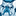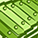# QlikView App Dev

Discussion Board for collaboration related to QlikView App Development.

Announcements
Become an analytics expert with Qlik's new 15 week course: Applied Data Analytics using Qlik Sense. READ MORE
cancel
Showing results for
Did you mean:Contributor

## Set analysis and variables

I am trying to calculate market share by date and facility name for my dataset. I utilizing a mix of static and dynamic dimensions. In my dimensions I have eTimeSelection_TopN and facility.name (in that order).

eTimeSelection_TopN =

if(max(total if(GetFieldSelections(freq) = 'Year + Quarter',r.idx,yr.idx2)) - if(GetFieldSelections(freq) = 'Year + Quarter',r.idx,yr.idx2) < if(GetFieldSelections(freq) = 'Year + Quarter',8,5),if(GetFieldSelections(freq) = 'Year + Quarter',full.abv,display.txt))

• r.idx and yr.idx2 are integers that indicate date order
• full.abv and display.txt are the static dimension that are interchanged based on the user's selection in a list box.

My expression looks like this : sum( <facility.name, \$(=eTimeSelection_TopN)> measure) /  sum(<\$(=eTimeSelection_TopN)> measure).

Nothing is returned by expression and in the caption title of the straight table it displays "Error: Error in expression". Any thoughts as to what might be going on? If I change the expression to sum(measure) it calculates and displays correctly.

6 RepliesMaster II

May be as below:

sum( <facility.name, \$('eTimeSelection_TopN')> measure) /  sum(<\$('eTimeSelection_TopN')> measure).

OR

sum( <facility.name, \$(eTimeSelection_TopN)> measure) /  sum(<\$(eTimeSelection_TopN)> measure).

OR

sum( <facility.name, '\$(eTimeSelection_TopN)'> measure) /  sum(<'\$(eTimeSelection_TopN)'> measure).Contributor
Author

Neither worked. I get the same as before. What is the intent of changing the order of the ' ?MVP

You may need TOTAL qualifier like

sum(TOTAL <facility.name, \$(=eTimeSelection_TopN)> measure) /  sum(TOTAL <\$(=eTimeSelection_TopN)> measure).

Before develop something, think If placed (The Right information | To the right people | At the Right time | In the Right place | With the Right context)MVP

Hi Ryan,

Add single quotes around the field names. Try exactly what I have mentioned below.

Variable name:

eTimeSelection_TopN

Definition:

=if(max(total if(GetFieldSelections(freq) = 'Year + Quarter',r.idx,yr.idx2)) - if(GetFieldSelections(freq) = 'Year + Quarter',r.idx,yr.idx2) < if(GetFieldSelections(freq) = 'Year + Quarter',8,5),if(GetFieldSelections(freq) = 'Year + Quarter','full.abv','display.txt'))

Expression:

sum( <facility.name, \$(eTimeSelection_TopN)> measure) /  sum(<\$(eTimeSelection_TopN)> measure)

By the way, I am not sure whether the formula which you showed is correct. There is no else part in your condition. If the calculation is less than, then the below line will be considered and you will see field names. If it's greater than, then you will see only hyphen since you haven't mentioned any else part. Hop you already aware this point.

if(GetFieldSelections(freq) = 'Year + Quarter','full.abv','display.txt')Contributor
Author

Anil: still same error message.

Tamil : The solution did not work. Changing to single quotes around the fields replaced the contents of the field with field name. The results went from showing "Q2-12-Q1'13" to "display.txt".

set vTopN = if(GetFieldSelections(freq) = 'Year + Quarter',8,5);
set eTimeField = if(GetFieldSelections(freq) = 'Year + Quarter',full.abv,display.txt);
set eTimeIndex = if(GetFieldSelections(freq) = 'Year + Quarter',r.idx,yr.idx2);
set eTimeSelection_TopN = if(max(total \$(eTimeIndex)) - \$(eTimeIndex) < \$(vTopN),\$(eTimeField));

The field freq is manipulated through the user's selection in a list box. The variable definition that I posted previously gets expanded in the variable overview window after the script is loaded.MVP

Hi Ryan,

Sorry for the late reply. I'm not sure what you want to see there. You want to show field name or value? What I have understood is you wanted to include the dimension names dynamicay based on the user selection. Correct me If I am wrong. I would suggest you to paste all those variables in text boxes and test yourself whether its showing expected result or not. This way you can identify which variable is creating problem. Let us know.Community Browser